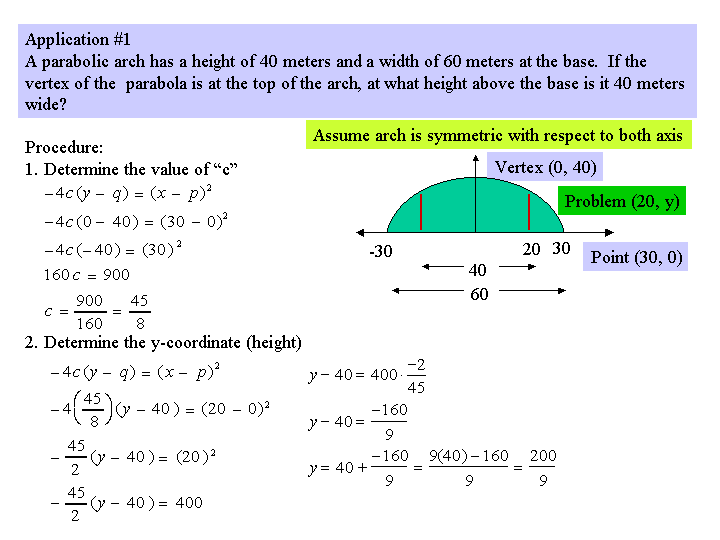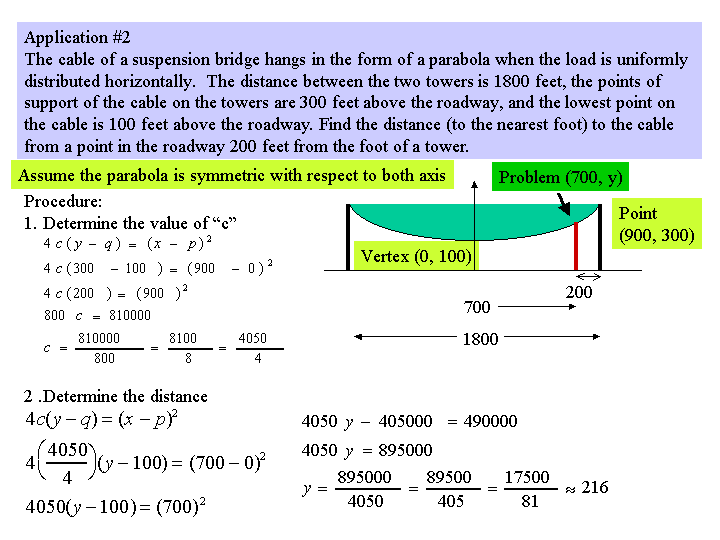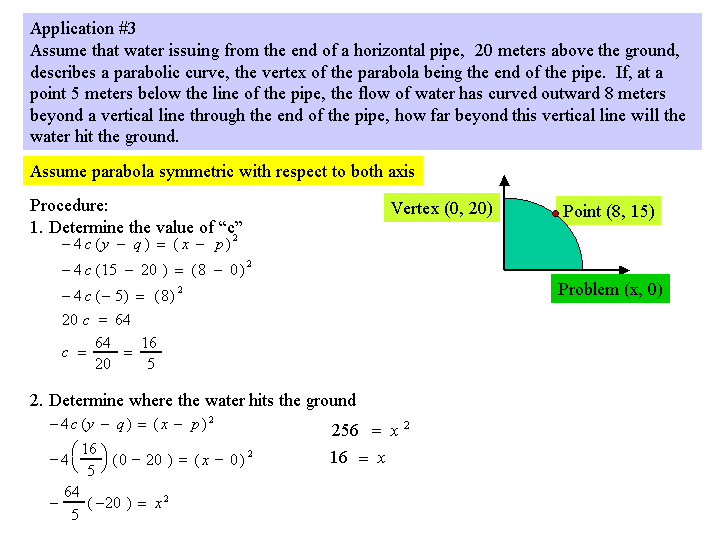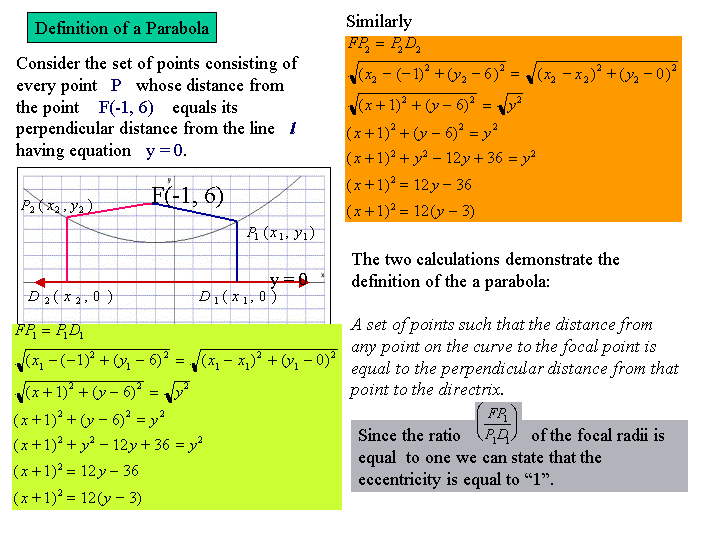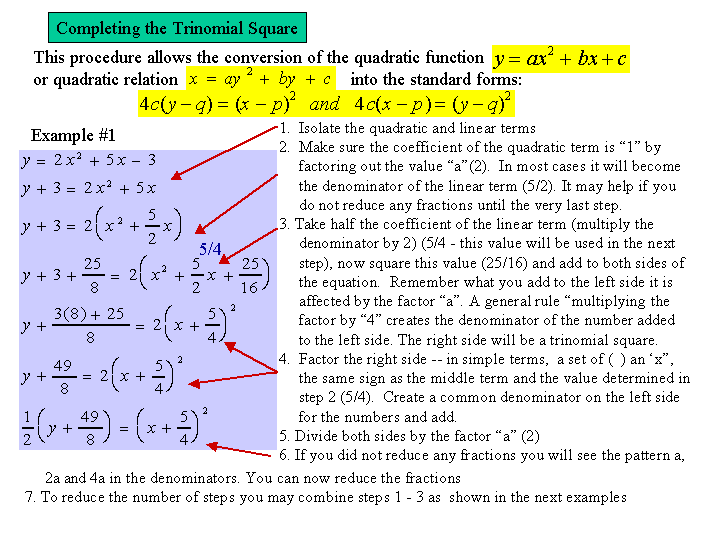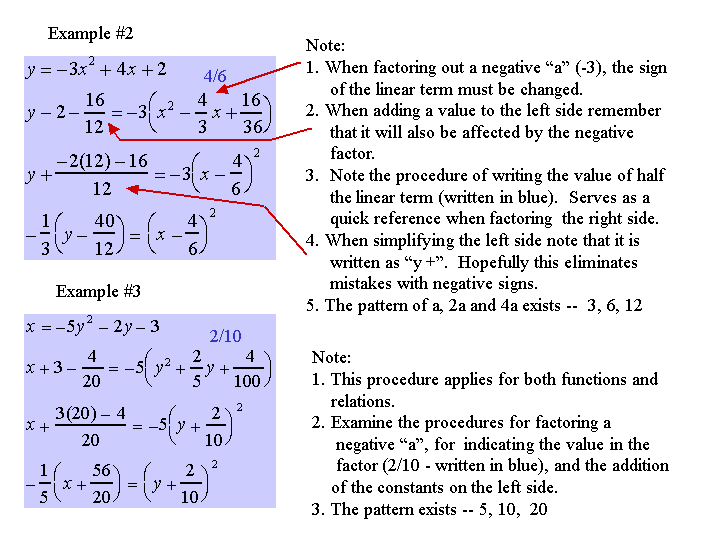If you what to practice the process of completing the trinomal sqaure use the worksheet Parabola - Characteristics From An Equation.(a pdf file). The general form and standard form are provided.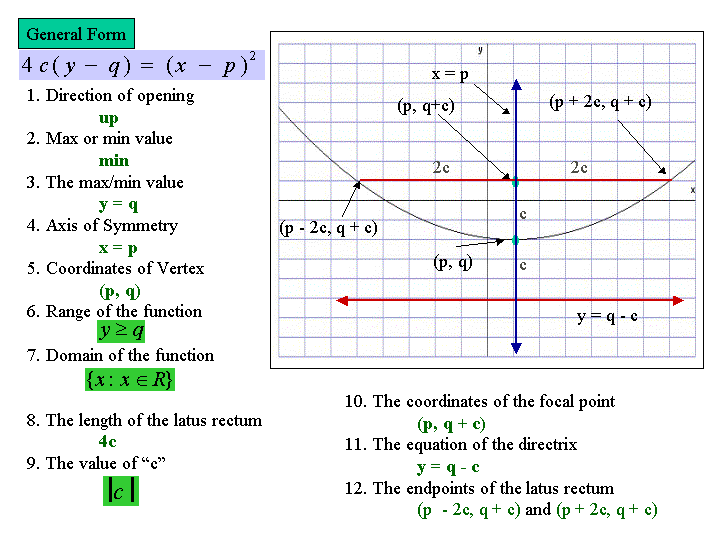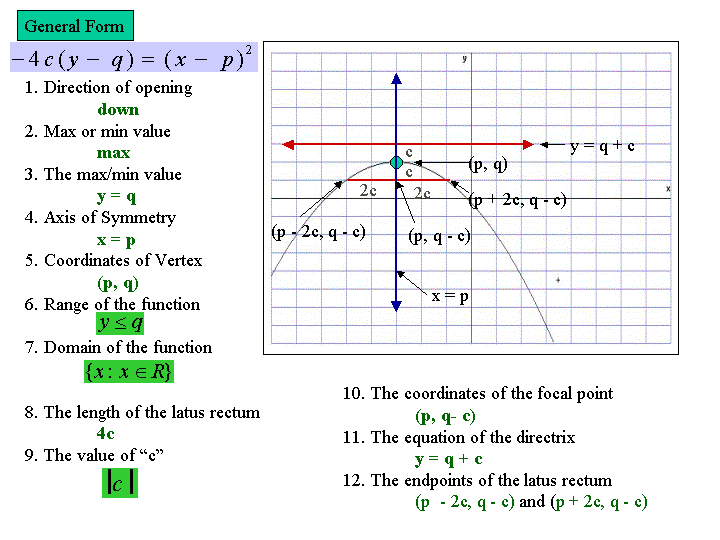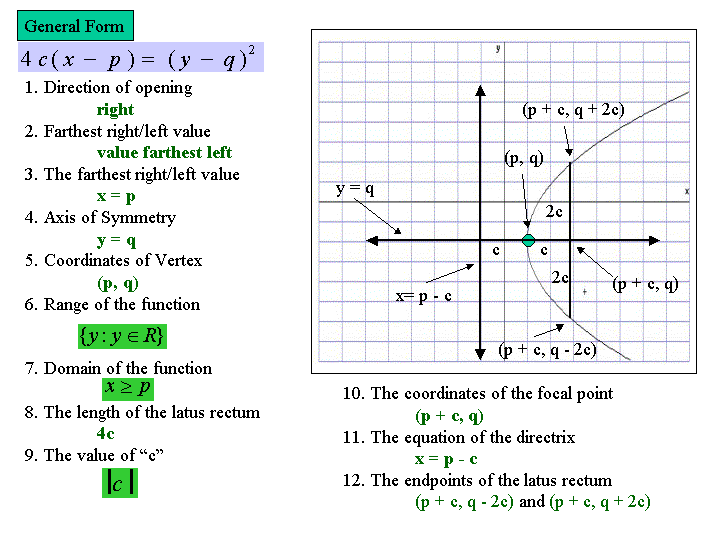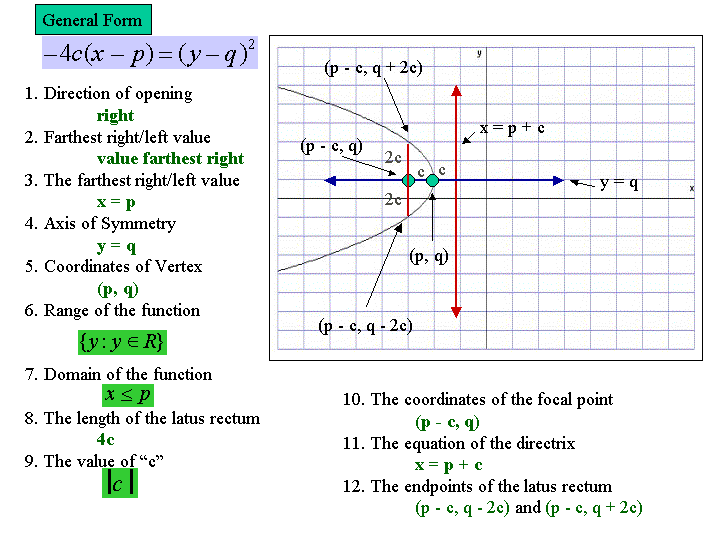Use the worksheet Parabola - Characteristics From An Equation (a pdf file) and Parabola Template for Characteristics to develop your skills for breaking a parabola in standard form into its component parts.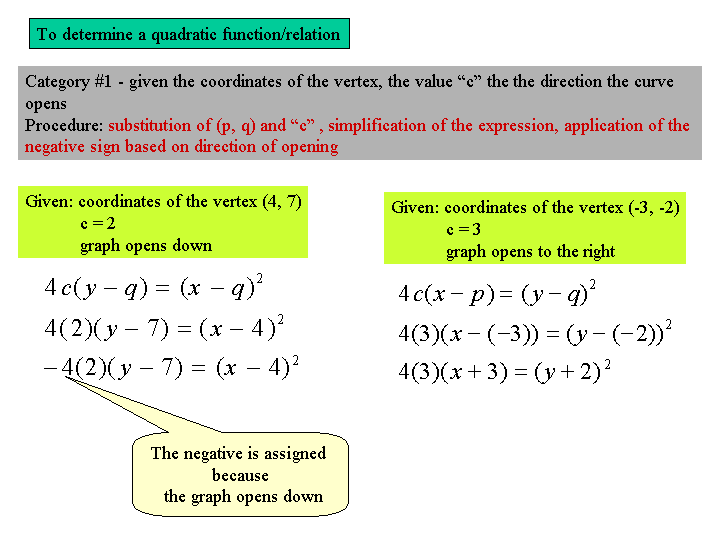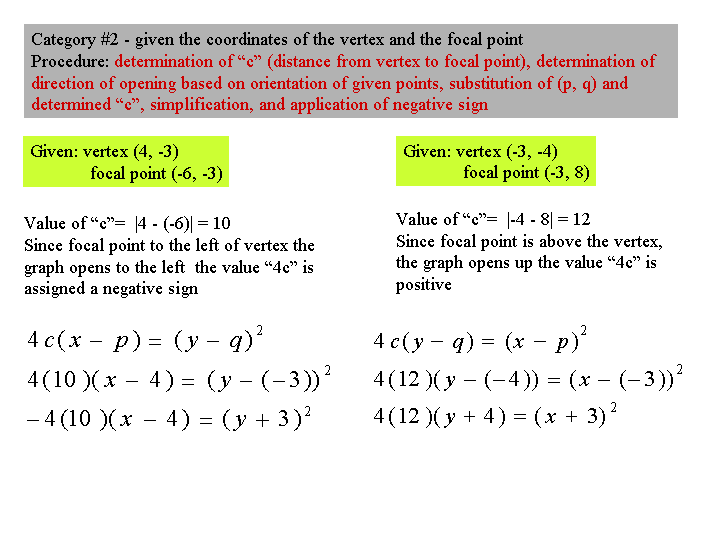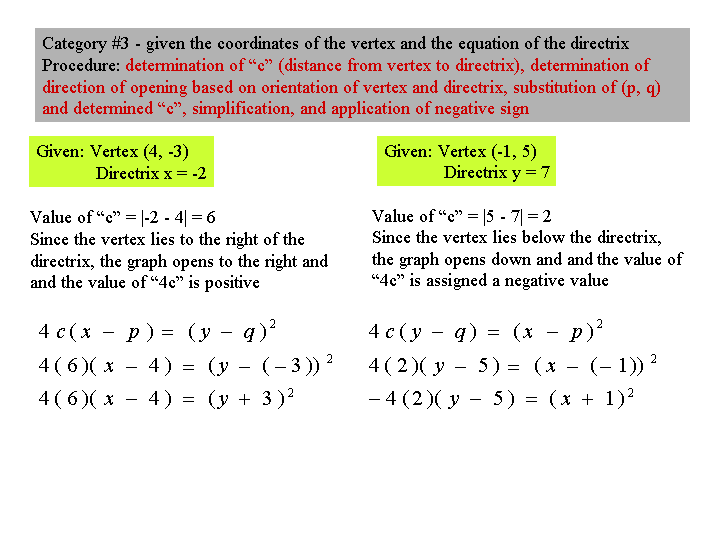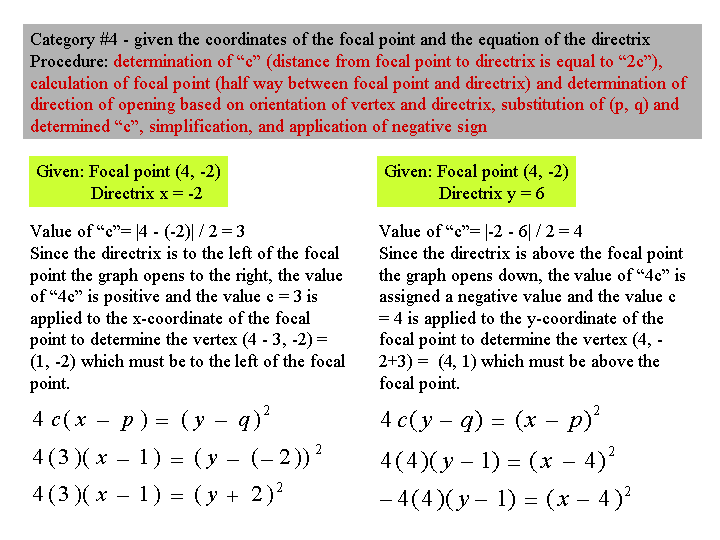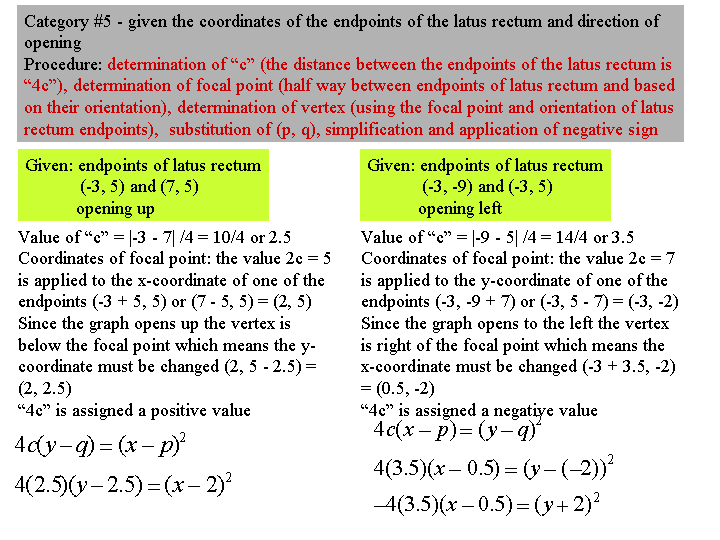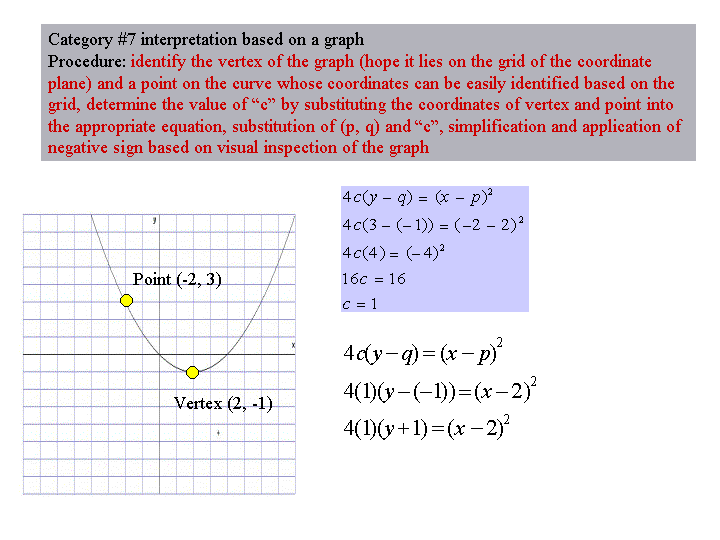Use the following assignments Parabola Build Up and Quadratic Function Graphs to practice your skillls in building an equation into standard form